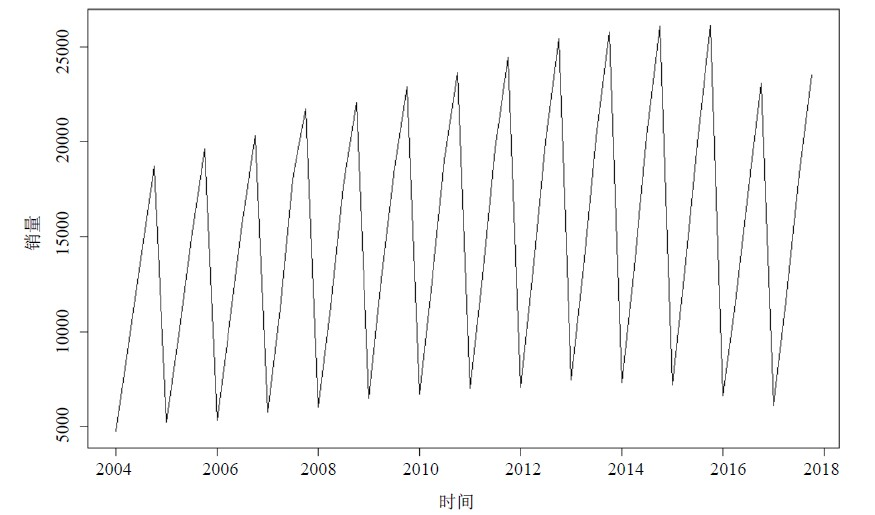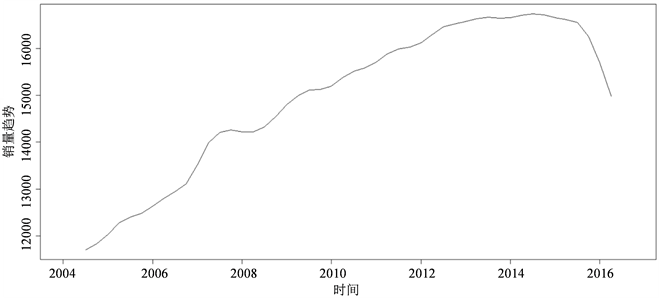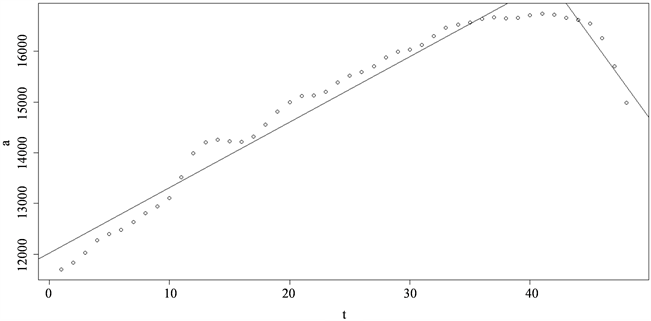# 基于时间序列模型的全国卷烟销量分析Analysis of National Cigarette Sales Based on Time Series Model

• 全文下载: PDF(629KB)    PP.1974-1981   DOI: 10.12677/ASS.2018.712293
• 下载量: 130  浏览量: 1,060

Accurate prediction of cigarette sales can provide guarantee for cigarette companies to formulate scientific marketing strategies. This article selects the national cigarette sales quarterly sales data from first quarter of 2004 to fourth quarter of 2016, by using the time sequence of certainty factor decomposition model to analyze its trend characteristics and seasonal fluctuations, and fits out the national cigarette sales model. The results are better, which provides a better model basis for the subsequent prediction of cigarette sales.

1. 研究背景

2. 国内研究现状

3. 理论模型选择

3.1. 时间序列分解模型

3.2. 移动平均法

${\stackrel{^}{x}}_{t}\left(1\right)=\frac{\left({x}_{t}+{x}_{t-1}+\cdots +{x}_{t-n-1}\right)}{n}$

${\stackrel{^}{x}}_{t}\left(2\right)=\frac{\left({\stackrel{^}{x}}_{t}\left(1\right)+{x}_{t}+\cdots +{x}_{t-n-2}\right)}{n}$

${\stackrel{^}{x}}_{t}\left(l\right)=\frac{\left({\stackrel{^}{x}}_{t}\left(l-1\right)+\cdots +{\stackrel{^}{x}}_{t}\left(1\right)+{x}_{t}+\cdots +{x}_{t-n-1}\right)}{n}$

3.3. 季节指数

4. 数据来源及处理Table 1. The first quarter of 2004 to 2016 in the fourth quarter of the national cigarette sales quarterly sales dataFigure 1. Line: The first quarter of 2004 to 2016 in the fourth quarter of the national cigarette sales quarterly sales data

5. 全国卷烟销量时间序列分解模型

5.1. 趋势变动分析Table 2. Resulting data of moving averageFigure 2. Curve: national cigarette sales trend

$T=\left\{\begin{array}{c}12020.178+129.335tt\le 42\\ 32853.11-368.33tt\ge 43\end{array}$Figure 3. Scatter plot and fitting line after moving average

5.2. 季节效应分析Table 3. Resulting data of seasonal index

5.3. 模型的拟合

${X}_{t}={T}_{t}\cdot {S}_{t}\cdot {I}_{t}=\left\{\begin{array}{c}\left(12020.178+129.335t\right)\cdot {S}_{t}t\le 42\\ \left(32853.11-368.33t\right)\cdot {S}_{t}t\ge 43\end{array}$

6. 结论Table 4. Comparison of historical data with predicted values

  高洪利. 基于随机森林算法的卷烟销量预测及分析[C]//中国烟草学会. 2017年中国烟草学会学术年会优秀论文集. 北京: 中国烟草学会, 2017: 1958-1968.  邹亮. 基于组合预测模型的怀化卷烟需求预测分析[J]. 中国烟草学报, 2014, 15(2): 80-85.  朱俊江, 何湘竹, 王建树, 李孝禄, 张远辉. 基于混合模型的卷烟销售量预测[J]. 中国烟草学报, 2016, 22(5): 111-116.  王伟民, 汪沄, 张国安. 基于灰色马尔科夫模型的全国卷烟需求预测研究[J]. 中国烟草学报, 2009, 15(6): 66-69.  曹鲁东. 卷烟销量应用研究——基于时间序列分析[D]: [硕士学位论文]. 昆明: 云南财经大学, 2013.  王燕. 时间序列分析基于R [M]. 北京: 中国人民大学出版社, 2015.  贾俊平. 统计学[M]. 北京: 清华大学出版社, 2006.